# Transient Response of a Spring-mass System with Imposed AccelerationThis test lets you check analysis results for a spring-mass system, in the context of a transient dynamic response case. You will use a 1D mesh.

This test is used to validate the following attributes:

• 1D beam element

• Transient dynamic response solve algorithms.Reference:

Guide de validation des prologiciels de calcul de structures, AFNOR Technique, SFM, pp182-183

##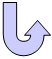Specifications

### Geometry Specifications

 Mass: m = 1 kg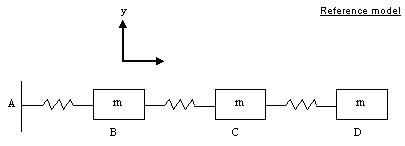Stiffness: k = 1000 N_m Length: L=1 m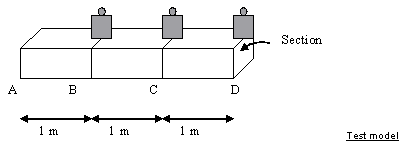Section: area = 1.10-5 m2

### Analysis Specifications

 Young Modulus (material): E = 100 MPa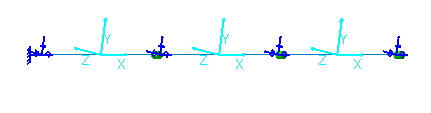Mesh Specifications: Size = 187.5 mm Restraints: Clamp on A Ty = Tz = Rx = Ry = Rz = 0 for each beam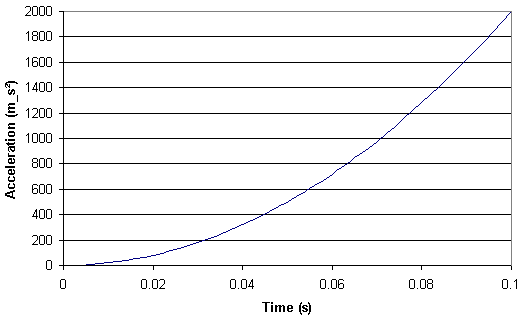Loads: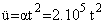0 s < t < 0.1 s Damping: d = 0 %

##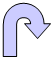Results

### Analytical Solution

The natural frequencies are the roots of the following equation: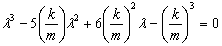The response at point D is given by: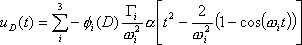with imposed acceleration coefficient: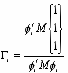and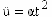modal participated factor for a translational motion with u = 1.

### Frequency Analysis

 Mode Frequencies [Hz] Error [%] Analytic solution Values 1 2.239 2.2399 0.038 2 6.275 6.2760 0.015 3 9.069 9.0690 0

### Transient Dynamic Analysis:

 Time [s] Displacement [mm] Error [%] Analytic solution Values 0.02 2.7 2.69 0.25 0.04 42.6 42.77 0.40 0.05 104.1 104.32 0.21 0.06 215.8 216.18 0.18 0.08 681.3 682.16 0.13 0.1 1658 1659.71 0.10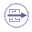To Perform the Test:

The Transient_response_spring_mass_system.CATAnalysis document presents a complete analysis of this case.

Proceed as follow:

1. Open the CATAnalysis document.

2. Compute the case and generate an image called Deformed mesh.